# plot

Plot parameter confidence interval results

## Syntax

``fh = plot(paraCI)``
``fh = plot(paraCI,Name,Value)``

## Description

example

````fh = plot(paraCI)` plots confidence intervals from `paraCI`, a `ParameterConfidenceInterval` object or vector of objects. If the estimation status of a confidence interval (`paraCI.Results.Status`) is `success`, the `plot` function uses the first default color (blue) to plot a line and a centered dot for every parameter estimate. The function also plots a box to indicate the confidence intervals.If the status is `constrained` or `estimable`, the function uses the second default color (red) and plots a line, centered dot, and box to indicate the confidence intervals. If the status is `not estimable`, the function plots only a line and a centered cross in red.If there are any transformed parameters with estimated values that are 0 (for the `log` transform) and 0 or 1 (for the `probit` or `logit` transform), no confidence intervals are plotted for those parameter estimates. ```
````fh = plot(paraCI,Name,Value)` uses additional options specified by one or more `Name,Value` pair arguments.```

## Examples

collapse all

Load the sample data to fit.

```load data10_32R.mat gData = groupedData(data); gData.Properties.VariableUnits = {'','hour','milligram/liter','milligram/liter'}; sbiotrellis(gData,'ID','Time',{'CentralConc','PeripheralConc'},'Marker','+',... 'LineStyle','none'); ```Create Model

Create a two-compartment model.

```pkmd = PKModelDesign; pkc1 = addCompartment(pkmd,'Central'); pkc1.DosingType = 'Infusion'; pkc1.EliminationType = 'linear-clearance'; pkc1.HasResponseVariable = true; pkc2 = addCompartment(pkmd,'Peripheral'); model = construct(pkmd); configset = getconfigset(model); configset.CompileOptions.UnitConversion = true; ```

Define Dosing

Define the infusion dose.

```dose = sbiodose('dose','TargetName','Drug_Central'); dose.StartTime = 0; dose.Amount = 100; dose.Rate = 50; dose.AmountUnits = 'milligram'; dose.TimeUnits = 'hour'; dose.RateUnits = 'milligram/hour'; ```

Define Parameters

Define parameters to estimate.

```responseMap = {'Drug_Central = CentralConc','Drug_Peripheral = PeripheralConc'}; paramsToEstimate = {'log(Central)','log(Peripheral)','Q12','Cl_Central'}; estimatedParam = estimatedInfo(paramsToEstimate,... 'InitialValue',[1 1 1 1],... 'Bounds',[0.1 3;0.1 10;0 10;0.1 2]); ```

Fit Model

Perform an unpooled fit, that is, one set of estimated parameters for each patient.

```unpooledFit = sbiofit(model,gData,responseMap,estimatedParam,dose,'Pooled',false); ```

Perform a pooled fit, that is, one set of estimated parameters for all patients.

```pooledFit = sbiofit(model,gData,responseMap,estimatedParam,dose,'Pooled',true); ```

Compute Confidence Intervals for Estimated Parameters

Compute 95% confidence intervals for each estimated parameter in the unpooled fit using the Gaussian approximation.

```ciParamUnpooled = sbioparameterci(unpooledFit); ```

Plot the confidence intervals. If the estimation status of a confidence interval is `success`, it is plotted in blue (the first default color). Otherwise, it is plotted in red (the second default color), which indicates that further investigation into the fitted parameters might be required. If the confidence interval is `not estimable`, then the function plots a red line and centered cross. If there are any transformed parameters with estimated values that are 0 (for the log transform) and 1 or 0 (for the probit or logit transform), then no confidence intervals are plotted for those parameter estimates. To see the color order, type `get(groot,'defaultAxesColorOrder')`.

Groups are displayed from left to right in the same order that they appear in the `GroupNames` property of the object, which is used to label x-axis. The y-labels are the transformed parameter names.

```plot(ciParamUnpooled) ```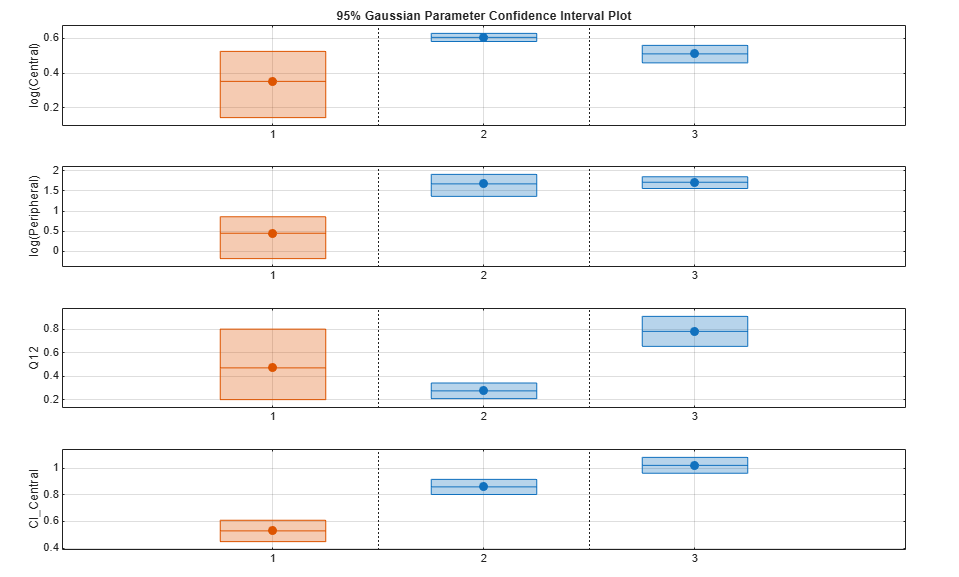Plot using a single color.

```plot(ciParamUnpooled,'Color',[0 0 0]) ```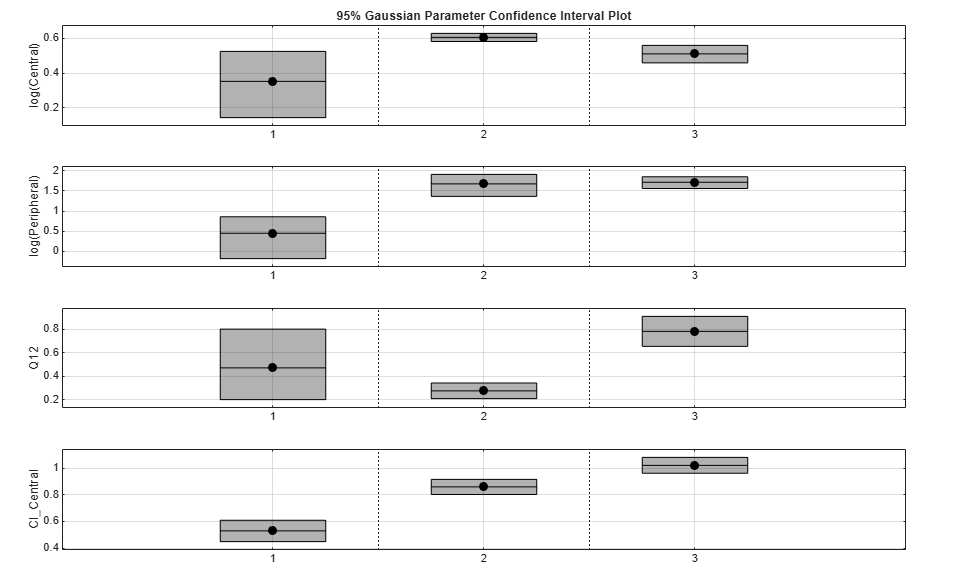Compute the confidence intervals for the pooled fit.

```ciParamPooled = sbioparameterci(pooledFit); ```

Plot the confidence intervals. The group name is labeled as "pooled" to indicate such fit.

```plot(ciParamPooled) ```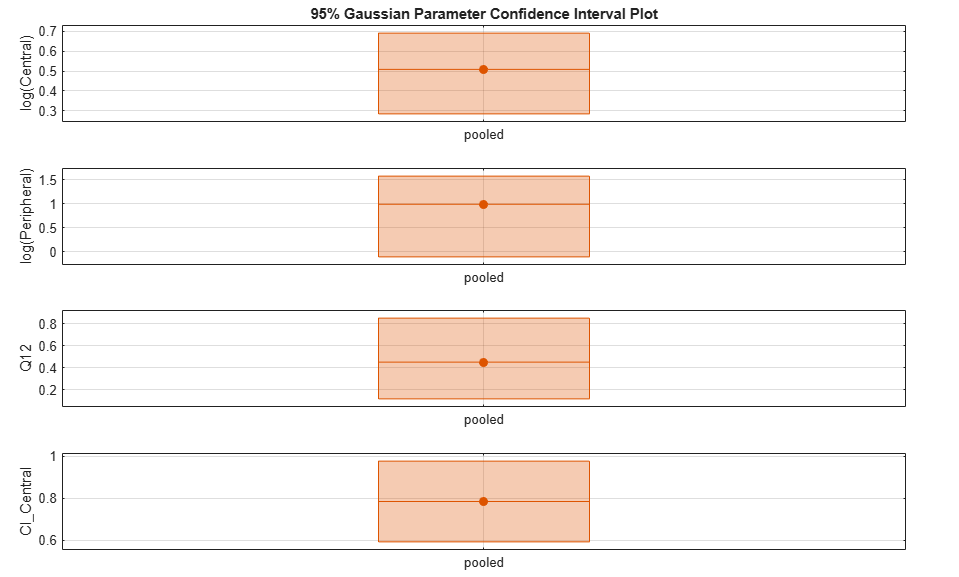Plot all the confidence interval results together. By default, the confidence interval for each parameter estimate is plotted in a separate axes. Vertical dotted lines group confidence intervals of parameter estimates that were computed in a common fit. Parameter bounds defined in the original fit are marked by square brackets (if visible in the parameter range being plotted).

```ciAll = [ciParamUnpooled;ciParamPooled]; plot(ciAll) ```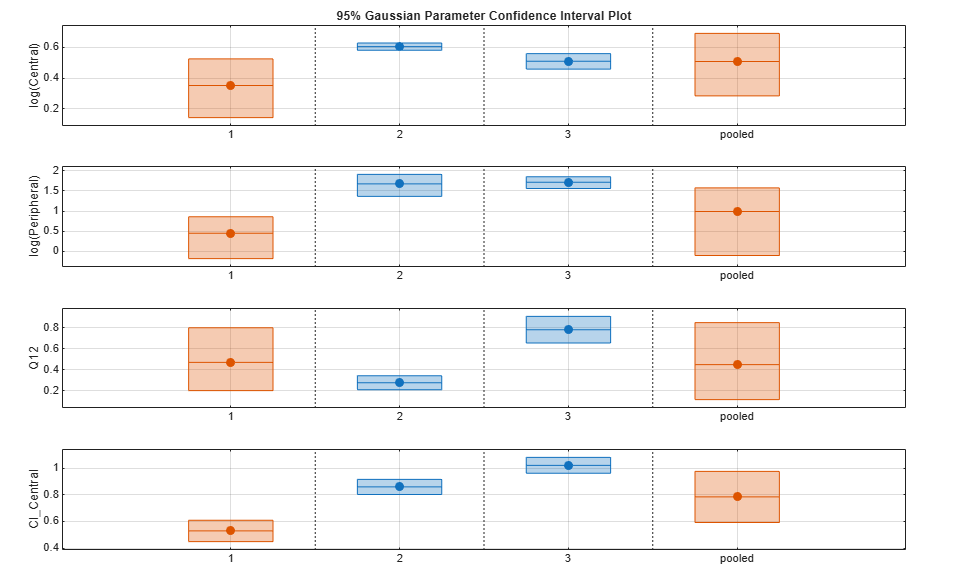You can also plot all confidence intervals on one axes grouped by parameter estimates using the 'Grouped' layout.

```plot(ciAll,'Layout','Grouped') ```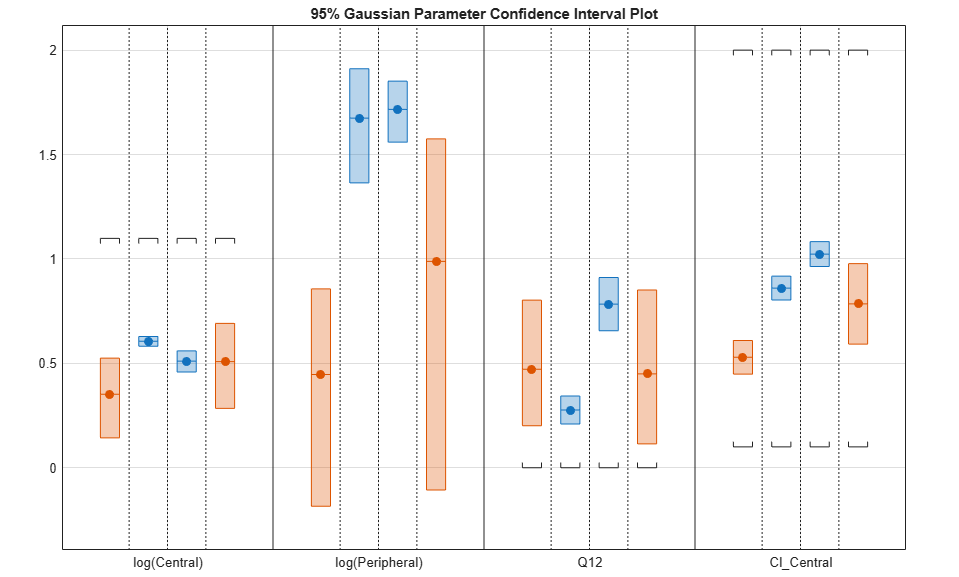In this layout, you can point to the center marker of each confidence interval to see the group name. Each estimated parameter is separated by a vertical black line. Vertical dotted lines group confidence intervals of parameter estimates that were computed in a common fit. Parameter bounds defined in the original fit are marked by square brackets (if visible in the parameter range being plotted). Note the different scales on the y-axis due to parameter transformations. For instance, the y-axis of `Q12` is in the linear scale, but that of `Central` is in the log scale due to its log transform.

Compute Confidence Intervals Using Profile Likelihood

Compute 95% confidence intervals for each estimated parameter in the unpooled fit using the profile likelihood approach.

```ciParamUnpooledProf = sbioparameterci(unpooledFit,'Type','profilelikelihood'); ```

Compute the confidence intervals for the pooled fit.

```ciParamPooledProf = sbioparameterci(pooledFit,'Type','profilelikelihood'); ```

Plot the profile likelihood curves for the unpooled fit. The parameter bounds defined in the original fit are displayed by vertical dotted lines (if visible in the parameter range being plotted). The confidence interval is indicated by two crosses and a line in between them. The center dot denotes the parameter estimate. The profile likelihood is always plotted in the log scale. The x-axis scale depends on whether the parameter is transformed (log, probit, or logit scale) or not (linear scale).

```plot(ciParamUnpooledProf,'ProfileLikelihood',true); ```Each group is plotted in a separate row, and each parameter is plotted in a separate column.

Plot the curves for the pooled fit.

```plot(ciParamPooledProf,'ProfileLikelihood',true); ```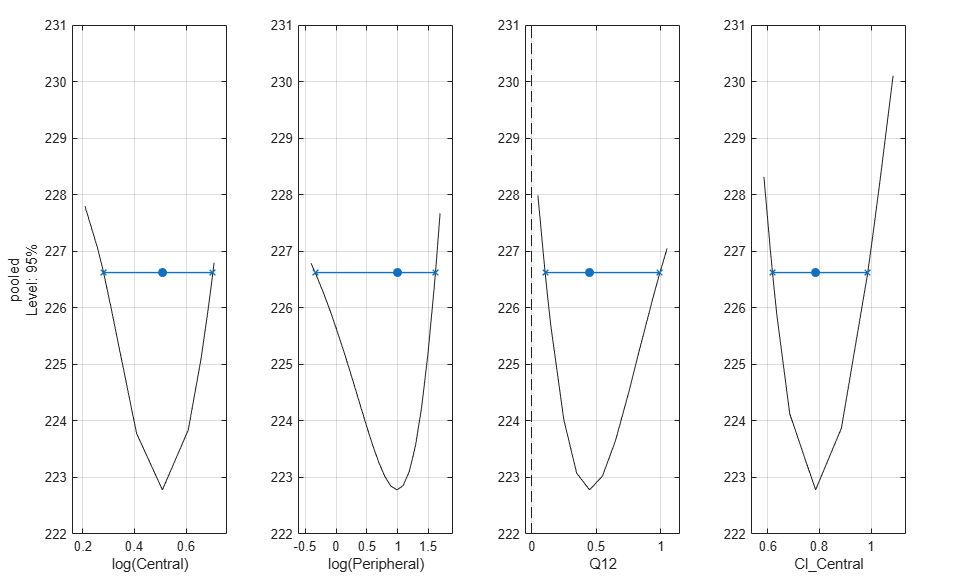Plot all the confidence interval results together in the same figure.

```plot([ciParamUnpooledProf;ciParamPooledProf],'ProfileLikelihood',true); ```## Input Arguments

collapse all

Parameter confidence interval results, specified as a `ParameterConfidenceInterval` object or a vector of objects.

### Name-Value Arguments

Specify optional pairs of arguments as `Name1=Value1,...,NameN=ValueN`, where `Name` is the argument name and `Value` is the corresponding value. Name-value arguments must appear after other arguments, but the order of the pairs does not matter.

Before R2021a, use commas to separate each name and value, and enclose `Name` in quotes.

Example: `'ProfileLikelihood',true` specifies to plot the profile likelihood curves.

Red-Green-Blue color triplet, specified as the comma-separated pair consisting of `'Color'` and a three-element row vector. By default, confidence intervals that are not limited by the parameter bounds specified in the original fit are plotted using the first default color (blue), and those that are limited by the bounds are plotted using the second default color (red). If the confidence interval is not estimable, it is also plotted in red. To see the default color order, enter `get(groot,'defaultAxesColorOrder')` or see the ColorOrder property.

Tip

Use this name-value pair when you want to create plots with a single color, for instance, for publication purposes.

Example: `'Color',[0 0 0]`

Logical scalar to display profile likelihood curves for the `profileLikelihood` confidence intervals, specified as the comma-separated pair consisting of `'ProfileLikelihood'` and `true` or `false`.

The confidence interval is indicated by two crosses with a line in between them. A center dot denotes the parameter estimate. The `plot` function uses the first default color (blue) for successfully computed confidence intervals. Otherwise, the function uses the second default color (red). A vertical dotted line marks the parameter bounds defined in the original fit.

If there are multiple groups, each group is plotted in a separate row and each parameter is plotted in a separate column. The labels for the x-axis are the transformed parameter names (the `TransformedName` property of the `estimatedInfo` object used in the original fit). The labels for the y-axis are the group names (the `GroupNames` property of the confidence interval object) and the confidence level.

The profile likelihood curve is always plotted in the log scale. The x-axis scale depends on whether the parameter is transformed (log, probit, or logit scale) or not (linear scale).

Example: `'ProfileLikelihood',true`

Axes layout to display parameter confidence intervals, specified as the comma-separated pair consisting of `'Layout'` and a character vector `'split'` (default) or `'grouped'`.

The `'split'` layout displays the confidence interval for each parameter estimate on a separate axes.

The `'grouped'` layout displays all confidence intervals on one axes grouped by parameter estimates. Each estimated parameter is separated by a vertical black line.

In both cases, the parameter bounds defined in the original fit are marked by square brackets. The function uses vertical dotted lines to group confidence intervals of parameter estimates that have been computed in a common fit.

Example: `'Layout','grouped'`

## Output Arguments

collapse all

Figure handle of the plot, returned as a figure handle.

## Version History

Introduced in R2017b